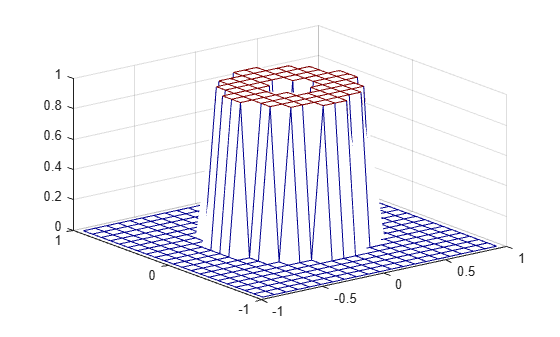# freqspace

Frequency spacing for frequency response

## Syntax

``[f1,f2] = freqspace(n)``
``[f1,f2] = freqspace([m n])``
``[x,y] = freqspace(___,'meshgrid')``
``f = freqspace(N)``
``f = freqspace(N,'whole')``

## Description

example

````[f1,f2] = freqspace(n)` returns the two-dimensional frequency vectors `f1` and `f2` for an `n`-by-`n` matrix. `freqspace` returns the implied frequency range for equally spaced frequency responses. `freqspace` is useful when creating desired frequency responses for various one- and two-dimensional applications.```
````[f1,f2] = freqspace([m n])` returns the two-dimensional frequency vectors `f1` and `f2` for an `m`-by-`n` matrix.```
````[x,y] = freqspace(___,'meshgrid')` is equivalent to [f1,f2] = freqspace(...); [x,y] = meshgrid(f1,f2); ```
````f = freqspace(N)` returns the one-dimensional frequency vector `f` assuming `N` evenly spaced points around the unit circle.```
````f = freqspace(N,'whole')` returns `N` evenly spaced points around the whole unit circle. In this case, `f` is `0:2/N:2*(N-1)/N`.```

## Examples

collapse all

Create a matrix `Hd` that contains the desired bandpass response for an approximately symmetric, two-dimensional bandpass filter with passband between 0.1 and 0.5 (normalized frequency, where 1.0 corresponds to half the sampling frequency, or π radians.) Use `freqspace` to create the frequency vectors `f1` and `f2`.

```[f1,f2] = freqspace(21,'meshgrid'); Hd = ones(21); r = sqrt(f1.^2 + f2.^2); Hd((r<0.1)|(r>0.5)) = 0; colormap(jet(64)) mesh(f1,f2,Hd)```## Input Arguments

collapse all

Length of the frequency vectors, specified as a positive numeric scalar.

Data Types: `single` | `double`

Length of each frequency vector, specified as a two-element vector of the form `[m n]`.

Data Types: `single` | `double`

Number of points around the unit circle, specified as a positive numeric scalar.

Data Types: `single` | `double`

## Output Arguments

collapse all

2-D frequency vector, returned as a numeric vector.

For `n` odd, both `f1` and `f2` are `[-n+1:2:n-1]/n`.

For `n` even, both `f1` and `f2` are `[-n:2:n-2]/n`.

2-D frequency vector, returned as a numeric vector. `f2` has the same odd and even behavior as `f1`.

X-coordinates over a grid, returned as a numeric matrix.

Y-coordinates over a grid, returned as a numeric matrix.

1-D frequency vector of points around the unit circle, returned as a numeric vector of `N` evenly spaced points.

• For `N` even or odd, `f` is `(0:2/N:1)`.

• For `N` even, `freqspace` returns `(N+2)/2` points.

• For `N` odd, `freqspace` returns `(N+1)/2` points.

## Version History

Introduced before R2006a